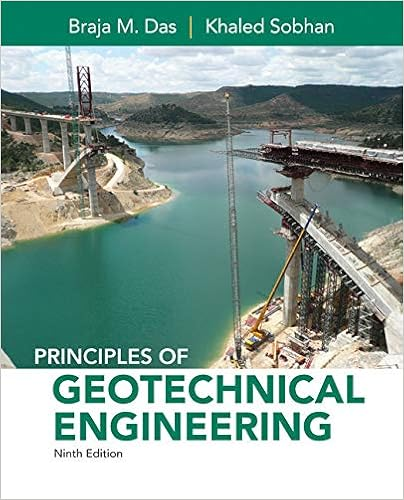# Previous answers a major league baseball pitcher

• Homework Help
• 16
• 78% (40) 31 out of 40 people found this document helpful

This preview shows page 9 - 13 out of 16 pages.

##### We have textbook solutions for you!
The document you are viewing contains questions related to this textbook.The document you are viewing contains questions related to this textbook.
Chapter 15 / Exercise 15.16
Principles of Geotechnical Engineering
Das/SobhanExpert Verified
9.6/6 points |Previous AnswersA major league baseball pitcher throws a pitch that follows these parametric equations:x(t) =140ty(t) = –16t2+5t + 5.The time units are seconds and the distance units are feet. The distance between the location of the pitcher and homeplate (where thebatter stands) is 60.5 feet. Give EXACT answers, unless instructed otherwise.(a) Calculate the horizontal velocity of the baseball at timet; this is the functionx'(t)=
(b) What is the horizontal velocity of the baseball when it passes over homeplate?
(c) What is the vertical velocity of the baseball at timet; this is the functiony'(t)=
(d) Recall that the speed of the baseball at timetiss(t)=√[x'(t)]2+ [y' (t)]2ft/sec.What is the speed of the baseball (inmph) when it passes over homeplate?
(e) At what time does the baseball hit the ground, assuming the batter and catcher miss the ball?
##### We have textbook solutions for you!
The document you are viewing contains questions related to this textbook.The document you are viewing contains questions related to this textbook.
Chapter 15 / Exercise 15.16
Principles of Geotechnical Engineering
Das/SobhanExpert Verified
sec.(f) What is themagnitudeof the angle at which the baseball hits the ground?
•••